Sale ends in
Teacher: Zinovy
Customers Who Have Viewed This Course: 2665
\$249.00
\$117.00

#### -1 Introduction to the course of Advanced Trigonometry 06:27

This course of Advanced Trigonometry encompasses all aspects traditionally included in studying of this subject :
Section 1. Introduction to Trigonometry
Section 2. Trigonometric Functions
Section 3. Trigonometric Identities
Section 4. Trigonometric Equations
Section 5. Trigonometric Inequalities
Section 6. Trigonometric Geometry
Section 7. Trigonometry and Complex Numbers
Section 8. Trigonometric Calculus

Each section contains certain number of video-recorded lessons, each with detail textbook-like description, which you can download and study off-line.
Most lessons are accompanied by multiple choice tests.

In addition, sections have exercises intended for you to apply your skills and knowledge to solve problems or prove theorems. In some cases these exercises contain answers for you to check your results. Important is to get these answers by yourself. Every such exercise has a subsequent review lesson, where these problems are analyzed in detail.

#### 0 Trigonometry 1.1. - Basic Concepts 16:35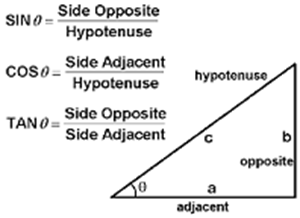This introductory lecture is about the history of Trigonometry, its main subject - the angles, ways to measure them, initial definitions of simple operations with angles and basic functions of them.

In particular, the definition of trigonometric functions for angles in right triangles is presented.

#### 1 Trigonometry 1.1. - Basic Concepts - Exercises 00:00

Important exercises on basic concepts of trigonometry - values of trigonometric functions for main types of angles of right triangles and construction problems on using elementary trigonometric functions.

#### 2 Trigonometry 1.1. - Basic Concepts - Exercises Review 16:35

This video reviews all exercises offered for lesson Trigonometry 1.1. - Basic Concepts

#### 3 Trigonometry 1.2. - Unit Circle 28:52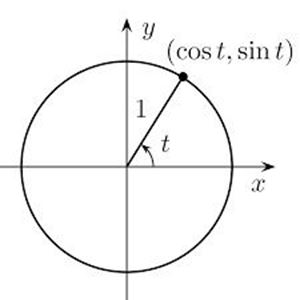This lecture introduces a concept of a unit circle on a coordinate plane.

Using this concept of a unit circle, we can define trigonometric functions SIN and COS for any angles, positive and negative, not limited to any range, measured in degrees or radians.

From functions SIN and COS we define all other trigonometric functions - TAN, COT, SEC and CSC.

We also demonstrate an equivalence of this definition of SIN and COS to a definition of these functions for angles in a right triangle as a ratio between its sides.

#### 4 Trigonometry 1.2. - Unit Circle - Exercises 00:00

Illustrative exercises on Unit Circle and Full Definition of Trigonometric Functions

#### 5 Trigonometry 1.2. - Unit Circle - Exercises Review 16:06

This video reviews all exercises offered for lesson Trigonometry 1.2. - Unit Circle

#### 6 Trigonometry 1.3. - Basic Angles 24:51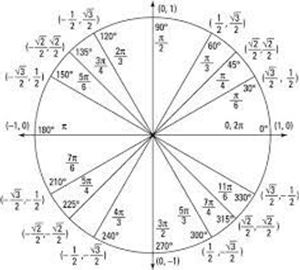Discussed methodology of derivation of the values of all trigonometric functions for most frequently occurred angles in trigonometric problems. This methodology has a simple foundation of a concept of a unit circle, Phifagorean Theorem and simple geometric properties of triangles.

#### 7 Trigonometry 1.3. - Basic Angles - Exercises 00:00

Values of trigonometric functions for angles outside the first quadrant.

#### 8 Trigonometry 1.3. - Basic Angles - Exercises Review 17:51

Values of trigonometric functions for angles outside the first quadrant.

#### 9 Trigonometry 1.4. - Simple Trigonometric Identities 27:30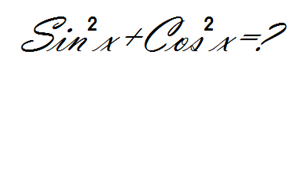We present a list of simple trigonometric identities with detailed proof of each.

#### 10 Trigonometry 1.4. - Simple Trigonometric Identities - Exercises 00:00

Based on a previous lecture, these exercises allow deeper understanding of relationship between trigonometric functions

#### 11 Trigonometry 1.4. - Simple Trigonometric Identities - Exercises Review 28:06

Review of exercises with simple trigonometric identities

#### 12 Trigonometry 1.5. - Right Triangles 23:27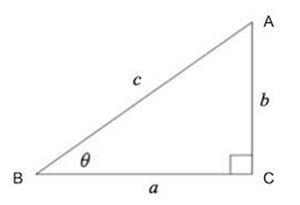Connection between sides, angles and trigonometric functions of these angles in right triangles:

In patricular, the following equalities are presented:

Equalities for a cathetus:
a = c·sin α
a = c·cos β
a = b·tan α
a = b·cot β
(with similar equalities for another cathetus)
Equalities for a hypotenuse:
c = a / sin α
c = a / cos β
(with similar equalities for another cathetus)

#### 13 Trigonometry 1.5. - Right Triangles - Exercises 00:00Solved are simple problems related to usage of trigonometric functions to determine characteristics of right triangles.

#### 14 Trigonometry 1.5. - Right Triangles - Exercises Review 11:45

Review of simple problems related to usage of trigonometric functions to determine characteristics of right triangles.

#### 15 Trigonometry 2.1. - Function y=SIN(x) 26:49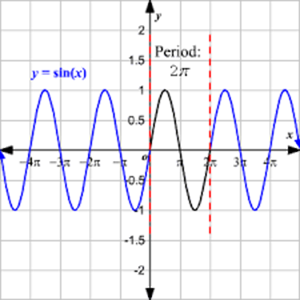Major properties of function y=SIN(x) are presented and proven. Discussed a graphical representation of this function.

#### 16 Trigonometry 2.2. - Function y=COS(x) 20:29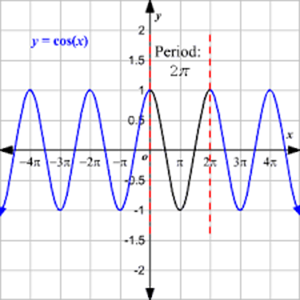Major properties of function y=COS(x) are presented and proven. Discussed a graphical representation of this function.

#### 17 Trigonometry 2.3. - Function y=TAN(x) 25:04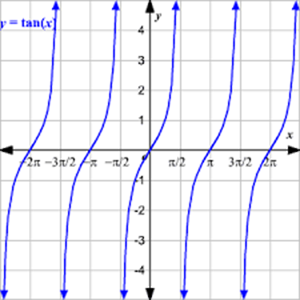Major properties of function y=TAN(x) are presented and proven. Discussed a graphical representation of this function.

#### 18 Trigonometry 2.4. - Function y=COT(x) 24:15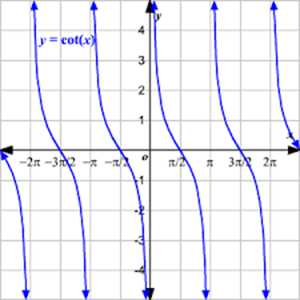Major properties of function y=COT(x) are presented and proven. Discussed a graphical representation of this function.

#### 19 Trigonometry 2.5. - Function y=SEC(x) 15:57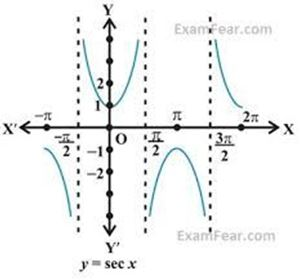Major properties of function y=SEC(x) are presented and proven. Discussed a graphical representation of this function.

#### 20 Trigonometry 2.6. - Function y=CSC(x) 16:58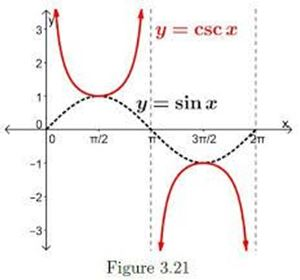Major properties of function y=CSC(x) are presented and proven. Discussed a graphical representation of this function.

#### 21 Trigonometry 2.7. - Trigonometric Functions - Properties - Exercises 00:00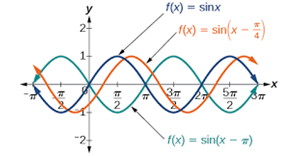For each of six trigonometric functions (SIN, COS, TAN, COT, SEC, CSC) answer the following questions:

A. Is it odd, even or neither?

B. Is it periodical and, if yes, what is the period?

C. Graph it.

D. What kind of symmetry, if any, does its graph have?

E. Does it equal to zero at some points and, if it does, where?

F. Does it have asymptotes and, if does, where?

G. What are its maximum and minimums and at what values of an argument?

#### 22 Trigonometry 2.7. - Trigonometric Functions - Properties - Exercises Review 27:41

Review exercises on properties of trigonometric functions

#### 23 Trigonometry 2.7. - Trigonometric Functions - Graphs - Exercises 00:00Exercises on graphic representation of trigonometric functions combined with linear graph transformation techniques

#### 24 Trigonometry 2.7. - Trigonometric Functions - Graphs - Exercises Review 27:41

Review of exercises on graphical representation of trigonometric functions

#### 25 Trigonometry 2.8. - Inverse Functions 24:18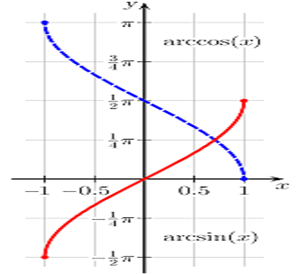In this lecture we introduce a general concept of an inverse functions, including the relationship between domain and range of an original function with domain and range of its inverse copunterpart. Also included the graphical representation af an inverse function.

#### 26 Trigonometry 2.8. - Inverse Trigonometric Functions - Definition 29:18Six inverse trigonometric functions (arcsin, arccos, arctan, arccot, arcsec, arccsc) are defined and analyzed, including their domains, ranges, properties and graphs.

#### 27 Trigonometry 2.8. - Inverse Trigonometric Functions - Exercises 00:00Exercise on inverse trigonometric functions cover the following three issues for each such function:

1. What are the domain, co-domain (range) of a particular inverse trigonometric function?
2. Draw a graph of this function.
3. What are the values of this function for certain specific values of argument?

#### 28 Trigonometry 2.8. - Inverse Trigonometric Functions - Exercises Review 29:18

Review of exercises on properties of inverse trigonometric functions

#### 29 Trigonometry 3.1. - Basic Identities 28:05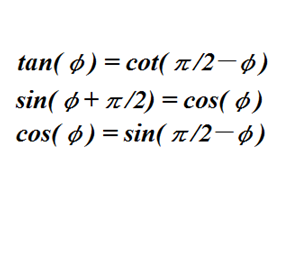Presented and rigorously proven are a few basic trigonometric identities. In particular, simple correspondence between sin(x) and cos(x), trivial for acute angles, is expanded to all angles.

#### 30 Trigonometry 3.2. - Formula for cos(2x) 16:52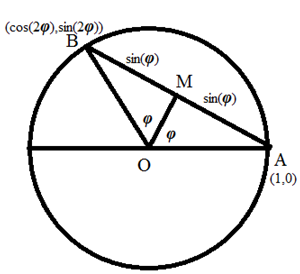Derived and proved for all types of angles a formula for cos(2x) in terms of cos(x) and/or sin(x)

#### 31 Trigonometry 3.3. - Formula for cos(x+y) 27:33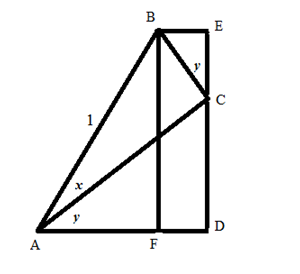In this lecture we present a proof of formula cos(x+y)=cos(x)cos(y)-sin(x)sin(y). First, geometrically, for acute angles, and then expanded to all angles in one particular case (other cases are analogous) using basic identities presented earlier in the course.

Also presented is a geometric proof for formula cos(x-y)=cos(x)cos(y)+sin(x)sin(y) for acute angles. Expansion to any angles is similar to above.

#### 32 Trigonometry 3.4. - Other Formulas for (x+y) 19:38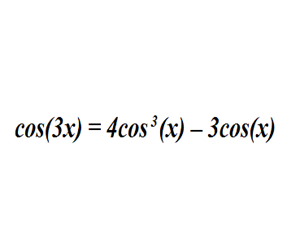Formulas for sin, tan, cot of a sum of or a difference between angles are presented in this lecture.

Also, formulas for sin and cos of a tripple angle are addressed.

All formulas are rigorously proven.

#### 33 Trigonometry 3.5. - Exercises with Identities - Part 1 00:00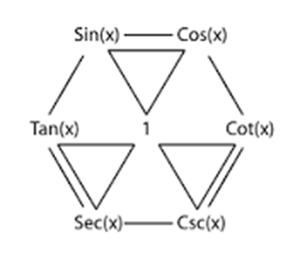Theese exercises are intended as an illustration of techniques used in trigonometry to simplify and transform trigonometric expressions and to assist in developing the logic behind these manipulations.

#### 34 Trigonometry 3.5. - Exercises with Identities - Part 1 - Review 21:31

Review exercises that are intended as an illustration of techniques used in trigonometry to simplify and transform trigonometric expressions

#### 35 Trigonometry 3.5. - Exercises with Identities - Part 2 00:00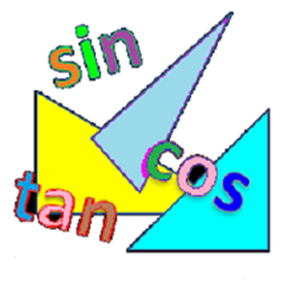Two conditional identities are presented that require some technical skills to be proven

#### 36 Trigonometry 3.5. - Exercises with Identities - Part 2 - Review 22:48

Review of exercises with identities that are incrementally more involved, primarily on a technical side (need some accuracy in the proofs)

#### 37 Trigonometry 4.1. - Primitive Equations 27:05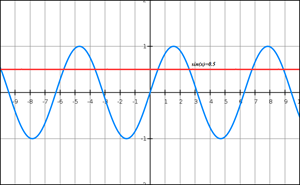Basic trigonometric equations of type sin(x)=a, tan(x)=a etc. are presented with discussion on methodology of solving them. Simple examples are provided as an illustration.

#### 38 Trigonometry 4.2. - Equations - Methodology 22:06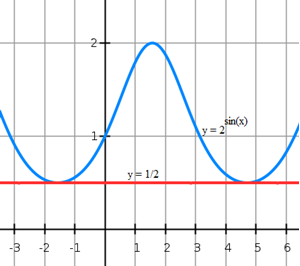Suggested methods to solve trigonometric equations for the following cases:

Linear transformation of an argument

Polynomial of a single trigonometric function

Function of a single trigonometric function

Equations with Mixed Trigonometric Functions

#### 39 Trigonometry 4.3. - Equations - Four Solutions 25:47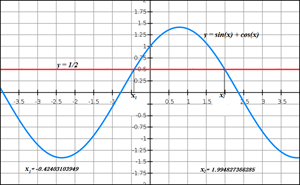A relatively simple equation is analyzed from four different viewpoints with four solutions looking differently, by numerically identical

#### 40 Trigonometry 4.4. - Equations - Exercises 1 00:00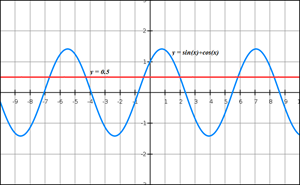A few problems with trigonometric equations are offered, explained, analyzed and solved

#### 41 Trigonometry 4.4. - Equations - Exercises 1 Review 29:22

Problems with trigonometric equations are explained, analyzed and solved.

#### 42 Trigonometry 4.4. - Equations - Exercises 2 00:00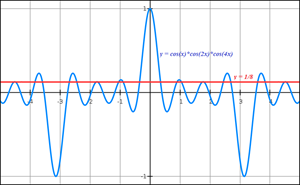A few more problems with trigonometric equations are presented, analyzed and solved.

#### 43 Trigonometry 4.4. - Equations - Exercises 2 Review 28:56

More problems with trigonometric equations are explained, analyzed and solved.

#### 44 Trigonometry 4.4. - Equations - Exercises 3 00:00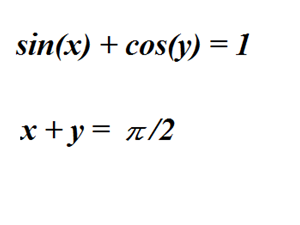Three examples of systems of two differential equations with two unknowns are presented to illustrate different methods of solving them.

#### 45 Trigonometry 4.4. - Equations - Exercises 3 Review 24:31

Problems with systems of trigonometric equations are explained, analyzed and solved.

#### 46 Trigonometry 5.1. - Inequalities - Overview 22:14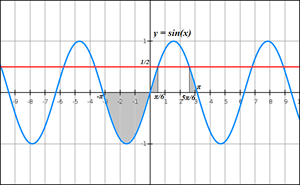Concept of trigonometric inequality and examples of primitive inequalities

tan(x) ≤ a,

cot(x) ≤ a,

sin(x) ≤ a and

cos(x) ≤ a

Emphasis on graphical interpretation of inequalities.

#### 47 Trigonometry 5.2. - Inequalities - Exercises 1 00:00

Presented are the following two problems:

Problem 1.1.

Solve the following inequality

sin(x)·sin(2x) + cos(x)·cos(2x) > sin(2x)

in the interval (π,π]

Problem 1.2.

Solve the following inequality

4·sin(x)·sin(2x)·sin(3x) > sin(4x)

(no restriction on argument x)

#### 48 Trigonometry 5.2. - Inequalities - Exercises 1 - Review 21:23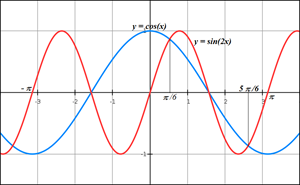Presented, analyzed and solved are a few problems with trigonometric inequalities.

#### 49 Trigonometry 5.2. - Inequalities - Exercises 2 00:00

Three more problems on trigonometric inequalities

#### 50 Trigonometry 5.2. - Inequalities - Exercises 2 - Review 24:54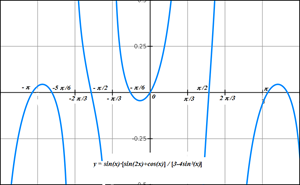These analyzed and solved problem on trigonometric inequalities introduce a couple of new techniques to solve them.

#### 51 Trigonometry 6.1. - Law of Sines 16:16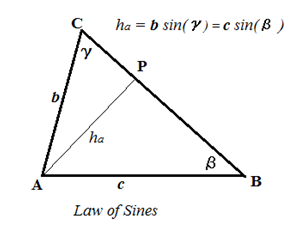Law of Sines is simple trigonometric identities that connect lengths of sides in a triangle with sines of opposite to these sides angles:

a/sin(α) = b/sin(β) = c/sin(γ)

#### 52 Trigonometry 6.2. - Law of Cosines 12:49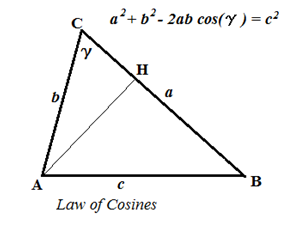Law of Cosines is an extension of Pythagorean Theorem to any triangle and connects the length of one side in a triangle with the lengths of two others and a cosine of an angle between them

a²+b²−2·a·b·cos(γ) = c²

#### 53 Trigonometry 6.3. - Geometrical Proof 17:44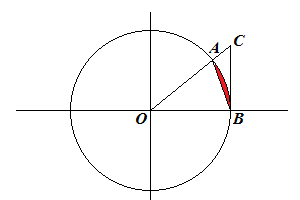Geometrical approach to prove important trigonometric inequalities:

sin(x) < x < tan(x) for x>0 and

cos(x) < sin(x)/x < 1

#### 54 Trigonometry 6.4. - Applications to Geometry - Exercises 1 00:00

Four geometrical problems are presented with solutions based on trigonometry.

#### 55 Trigonometry 6.4. - Applications to Geometry - Exercises 1 - Review 27:32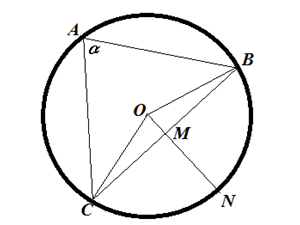Four geometrical problems are presented with solutions based on trigonometry. Among them are these (and two others)

Let be a point of intersection of altitudes in the triangle ΔABCProve that three circles that circumscribe triangles ΔABH, ΔBCHand ΔCAH have the same radius.

Express s(area of a triangle) in terms of R, α, β, γ.

#### 56 Trigonometry 6.4. - Applications to Geometry - Exercises 2 00:00

More problems that illustrate application of trigonometry to solving geometric problems

#### 57 Trigonometry 6.4. - Applications to Geometry - Exercises 2 - Review 22:18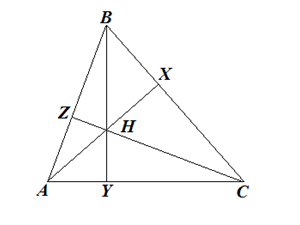1. Prove that for any triangle the following equality is true.
a·b·cos²(γ/2) = p·(p−c)

2. Prove that for any triangle the following equality is true.

a·b·cos²(γ/2) = p·(p−c)

3. Prove that the area of any triangle equals to

s = ¼·[a²·cot(α)+b²·cot(β)+c²·cot(γ)]

4. Prove that for any triangle the following equality is true.

8R³·ha·hb·hc=a²·b²·c²

#### 58 Trigonometry 6.4. - Applications to Geometry - Exercises 3 00:00

Four more Geometry problems that can be solved using Trigonometry.

#### 59 Trigonometry 6.4. - Applications to Geometry - Exercises 3 - Review 20:04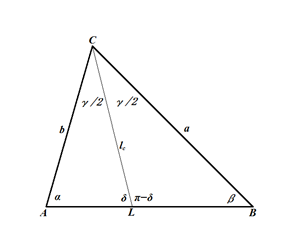Four more Geometry problems that can be solved using Trigonometry.

Among others, the following problem is offered.

Given a parallelogram ABCD with an acute angle ∠BAD=α.

#### 60 Trigonometry 7.1. - Complex Numbers 26:55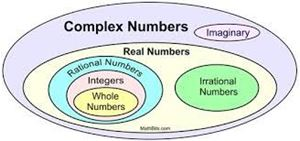The purpose of this lesson is not only to describe the expansion of a set of real numbers towards complex ones, but to justify the necessity of this and to introduce the complex numbers in a more rigorous fashion than traditionally presented to many students.

We will follow the evolution of a concept of number from natural to integer, to rational, to real and explain the necessity of this evolution in a quest for symmetry and harmony of our theory. That same quest will lead us to introduction of complex numbers.

Basic definitions that are used to create a new set of numbers are presented in details, as well as main operations with complex numbers and laws that direct these operations.

#### 61 Trigonometry 7.2. - Advanced Complex Numbers 27:50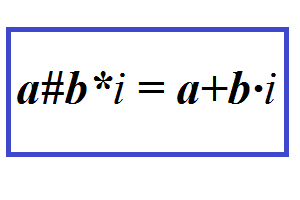This lesson is a more formal, abstract, more rigorous definition of complex numbers and operations with them.
The need for more rigorous definition of complex numbers lies in a simple fact that, defining any operation on two objects, we have to properly define a set these objects and the result of operation on them belong to.
If r is a real number and i is an imaginary unit, the square of which equals to -1 and is not a real number, what is the set they both belong to?
How to perform addition or multiplication on them?
What set the result of operation on them belongs to?

These and other questions can be answered by rigorously constructing a new set of complex numbers that properly represents all real numbers, all imaginary numbers and the results of operations on them. This is a subject of this lesson.

#### 62 Trigonometry 7.3. - Complex Trigonometry 23:46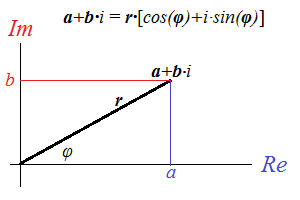Vector representation of complex numbers in Cartesian and polar coordinates.
Modulus and phase angle.
DeMoivre's formula.

#### 63 Trigonometry 7.4. - Complex Trigonometry - Exercise 00:00

An important trigonometric problem  that can be solved using the complex numbers and their representation in trigonometric form in polar coordinates

#### 64 Trigonometry 7.4. - Complex Trigonometry - Exercise - Review 23:11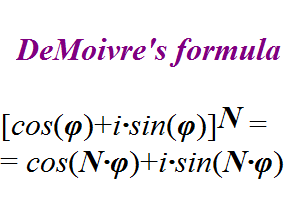In this lecture we will present an example of solving trigonometric problems using the presentation of complex numbers in trigonometric form.

Calculate the following sums using complexz = cos(x)+i·sin(x) and the Moivre's formula.
A = cos(x) + cos(2x) + cos(3x) + cos(4x) +...+ cos(Nx)

B = sin(x) + sin(2x) + sin(3x) + sin(4x) +...+ sin(Nx)

#### 65 Trigonometry 7.5. - Euler's Formula 23:07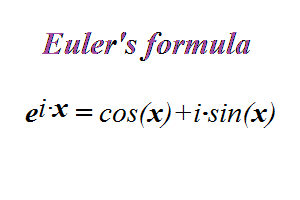Defining the operation of raising of a real number into the imaginary power (Euler's formula) and proving the validity of this definition.

#### 66 Trigonometry 7.5. - Euler's Formula - Exercises 00:00

A few problems related to applications of Euler's formula

#### 67 Trigonometry 7.5. - Euler's Formula - Exercises - Review 22:37

Analysis and solution of the few problems related to applications of Euler's formula

#### 68 Trigonometry 8.1. - Limit, Derivative, Integral 29:32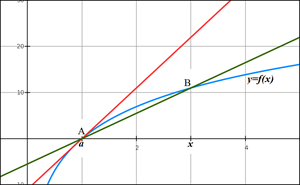Brief recap of main concepts of Calculus - Limits, Derivatives, Integrals - for the purpose of analyzing the behavior of trigonometric functions in subsequent lessons.

Definitions and main characteristics of these concepts are duscussed in a very short format.

#### 69 Trigonometry 8.2. - Calculus of Trigonometric Functions 29:00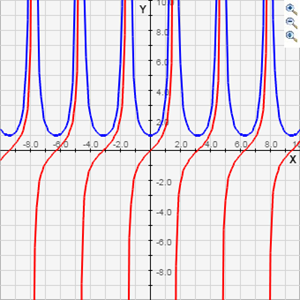Three functions, sin(x), cos(x) and tan(x) are analyzed using the tools of Calculus. In particular, the intervals of increasing and decreasing are identified as well as points of local maximum and minimum.

Learning is not just accumulation of information. Educational process, especially for such a subject as Mathematics, is an active two-way street. The teacher provides you with information and expects something in return – your full attention, participation, and effort, without which anything you get will soon be forgotten without any traces.
In return for your efforts you will be rewarded with Knowledge and develop your abilities to approach many new problems in a creative, analytical way, which leads to success in any field you will be working.

WHAT'S IN THE COURSE

This course of Advanced Trigonometry contains:

8 major sections dedicated to broad spectrum of topics of Trigonometry

30 video-recorded theoretical lessons distributed among these sections (up to 30 min each)

28 multiple choice students' tests with detail explanation of correct answers available as parents' resources

19 video-recorded practical exercise lessons that offer problems and their review (up to 30 min each)

Overall, more than 20 hours of video-recorded lessons!

IMPORTANT NOTES

Resources, available for supervising parents, contain answers to all multiple choice tests with detail reasoning why these answers are correct. These tests are very important and we encourage all parents to make sure that the answers provided by students are not only correct, but have logical reasoning behind them similar to considerations offered in answers provided in the resources.

Practical exercises are presented as two separate items each - a description and an explanation and analysis in the following lesson. They are intended for students to apply their skills and knowledge to solve problems or prove theorems by themselves and then check it against the explanation provided in the course. In some cases these exercises contain answers in the description itself to check the results. It is important to go through these answers prior to watching the subsequent lesson with full analysis and explanation.

COURSE GOAL

The primary goal of this course is to present the students with rigorous definitions, properties, relationships and dependencies among different objects studied in Trigonometry.

Perhaps the most interesting part of this course is the kind of problems offered as tests or exercises. They require not only factual knowledge of the information provided in theoretical lectures, but also certain degree of creativity, ingenuity and analytical thinking and, as such, are intended to develop these skills, which will be useful in any future profession. Development of these qualities in students is an extremely important goal of this course.

TARGET AUDIENCE

The course is intended for high school level students who are either involved in home schooling or are just interested in deepening their mathematical knowledge as a tool to develop their creativity, logic, analytical thinking and, arguably, general intelligence.

Those students who successfully complete this course will excel not only in Trigonometry, but in other mathematical topics and all aspects of their lives that involve deep understanding, thinking and decision making.

COURSE REQUIREMENTS AND PREREQUISITES

Strong algebraical and geometrical skills are assumed for students studying Trigonometry. In addition, knowledge of complex numbers, limits and derivatives would be useful. For those not familiar with the concepts of complex numbers, limits and derivatives the course contains introductory lectures, which introduces these concepts to a level sufficient to understand their application to Trigonometry.

COURSE TOPICS

This course of Advanced Trigonometry encompasses all aspects traditionally included in studying of this subject :

Section 1. Introduction to Trigonometry
(5 theoretical video lessons with detail notes and tests, 4 exercises with full review)
Basic Concepts
Unit Circle
Basic Angles
Simple Identities
Right Triangle

Section 2. Trigonometric Functions
(8 theoretical video lessons with detail notes and tests, 3 exercises with full review)
Properties of function y=SIN(x)
Properties of function y=COS(x)
Properties of function y=TAN(x)
Properties of function y=COT(x)
Properties of function 2.5 y=SEC(x)
Properties of function y=CSC(x)
Review of Properties of Trigonometric Functions
Inverse Trigonometric Functions

Section 3. Trigonometric Identities
(4 theoretical video lessons with detail notes and tests, 2 exercises with full review)
Basic Identities
Formula for cos(2x)
Formula for cos(x+y)
Other formulas for (x+y)

Section 4. Trigonometric Equations
(3 theoretical video lessons with detail notes and tests, 3 exercises with full review)
Primitive Equations
Methodology
Four Solutions to One Problem

Section 5. Trigonometric Inequalities
(1 theoretical video lesson with detail notes and tests, 2 exercises with full review)
Overview of Trigonometric Inequalities

Section 6. Trigonometric Geometry
(3 theoretical video lessons with detail notes and tests, 3 exercises with full review)
Law of Sines
Law of Cosines
Geometrical Proofs of Trigonometric Identities

Section 7. Trigonometry and Complex Numbers
(4 theoretical video lessons with detail notes and tests, 2 exercises with full review)
Introduction to Complex Numbers
Complex Trigonometry
Euler's Formula

Section 8. Trigonometric Calculus
(2 theoretical video lessons with detail notes and tests)
Limit, Derivative, Integral
Calculus of Trigonometric Functions

• Teacher: Zinovy
• Areas of expertise: Math, Computers, Finance
• Education: Moscow Lomonosov University, School of Mathematics
• Interests: Teaching, Politics
• Skills: Specialized in Theory of Probabilities and Mathematical Statistics
• Associations:
• Issues I care about: Education, Political Science, Technology, Health

My name is Zinovy (Zor). I am a mathematician by education, a computer programmer and a financial specialist by profession and a teacher by inspiration. I was born and educated in the Soviet Union, graduated as a mathematician from a prestigious Moscow University at the time when its math school was one of the best in the world. Though, I consider my high school years to be at least as important because of a brilliant math teacher who established a solid foundation to my future math study. After graduation from university I was working in the computer industry developing software for statistical applications. At age of 30 I with my family immigrated to the United States and continued my career in New York as a computer specialist primarily in the financial industry, working for the best in the world financial information provider and then for the largest asset management company in the world. I think, many of our high schools do not provide an adequate level of knowledge in such an important subject as Mathematics and Physics. I am sure, many young students, especially those studying these subjects at home, would welcome an opportunity to deepen their knowledge, while teachers might be benefited from a course concentrated on rigorous presentation of ideas, logic and methods. After retirement I have decided to dedicate my efforts to education to transfer my knowledge of Mathematics and system of study to those young students who appreciate rigorousness, logic and creativity and cannot get it in schools for any reason. When approached by Lernsys, I realized the potential of cooperation between this company, serving as a conduit of knowledge, and a provider of quality education to homeschoolers. I hope that the high quality of educational material I can provide, combined with publishing capabilities of Lernsys, allowing it to distribute these materials to a broad audience of students, will open a new page in the system of education.

#### Trigonometry - Answer Keys to Tests

Each document in this resource contains answers to corresponding test questions.